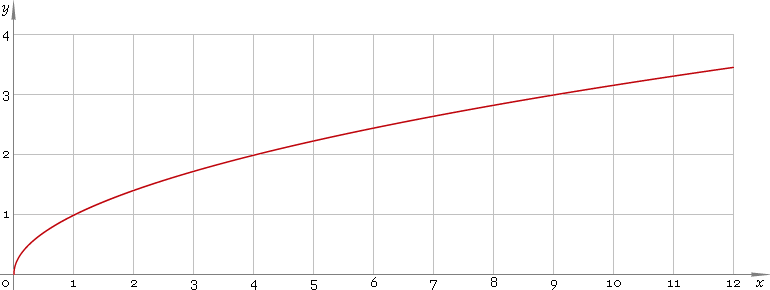The Art of Interface

# √ or sqrt — square root function

Category. Mathematics.

Abstract. Square root: definition, plot, properties and identities.

## 1. Definition

Square root function is inverse of the power function with power a = 2

x2

The square root is denoted with radical symbol:

x

Square root is equivalent to the power of one second:

xx1/2

## 2. Plot

Square root function defined for non-negative part of real axis — so, its domain is [0, +∞). Function plot is depicted below — fig. 1.Fig. 1. Plot of the square root function y = √x.

Function codomain non-negative part of the real axis: [0, +∞).

## 3. Identities

Take into account, that because of square root defined only for non-negative values, and power of two defined everywhere, the order of these two functions makes difference:

x2x
√(x2) ≡ |x|

and as well

x ≡ signx √(x2)

Reciprocal argument:

√(1/x) = 1 /√x

Product and ratio of arguments:

√(xy) = √|x|√|y|
√(x/y) = √|x| /√|y|

Power of argument:

√(xa) = √|x|a ≡ |x|a/2

## 4. Solution of quadratic equation

ax2 + bx + c = 0

has roots

x = [−b ± √(b2 − 4ac)] /(2a)

For equation with even coefficient for the first power ax2 + 2bx + c = 0

roots have simplified form

x = [−b ± √(b2ac)] /a

## 5. Solution of normalized quadratic equation

Normalized quadratic equation x2 + bx + c = 0

has roots

x = [−b ± √(b2 − 4c)] /2

And equation with even coefficient for the first power x2 + 2bx + c = 0

has the simplest form for its roots

x = −b ± √(b2c)

## 6. Support

Square root function or sqrt of the real argument is supported by free version of the Librow calculator.

Square root function or sqrt of the complex argument is supported by professional version of the Librow calculator.

## 7. How to use

To calculate square root of the number:

``sqrt(2);``

or

``√(2);``

To calculate square root of the current result:

``sqrt(rslt);``

or

``√(rslt);``

To calculate square root of the number x in memory:

``sqrt(mem[x]);``

or

``√(mem[x]);``